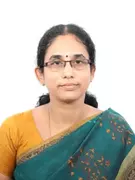X
Design of successive approximation (Sa) based low complexity multiplier-less coefficient decimation filter bank for sdr receivers
Published in Research Trend
2020
Volume: 11

Issue: 3
Pages: 959 - 964
Abstract
Coefficient decimation filter banks (CDFBs) found its application in software defined radio (SDR) channelizers because of its advantages such as highly reconfigurable and low complexity (number of multiplications). Multiplier-less realization is highly preferable when the ultimate goals are implementation simplicity and processing speed. This paper presents a design of multiplier-less CDFB where coefficient space of filters belongs to sum of powers-of-two (SOPOT) form. To obtain, the SOPOT approximations of impulse response values of the CDFB sub filters, a modified version of vector successive approximation (SA) technique termed as Matching Pursuits Generalized BitPlanes (MPGBP) algorithm is utilized. Design examples show that the computational time and implementation complexity (number of adders) of the proposed CDFB is lower than those of its counter parts. © 2020, Research Trend. All rights reserved.
•